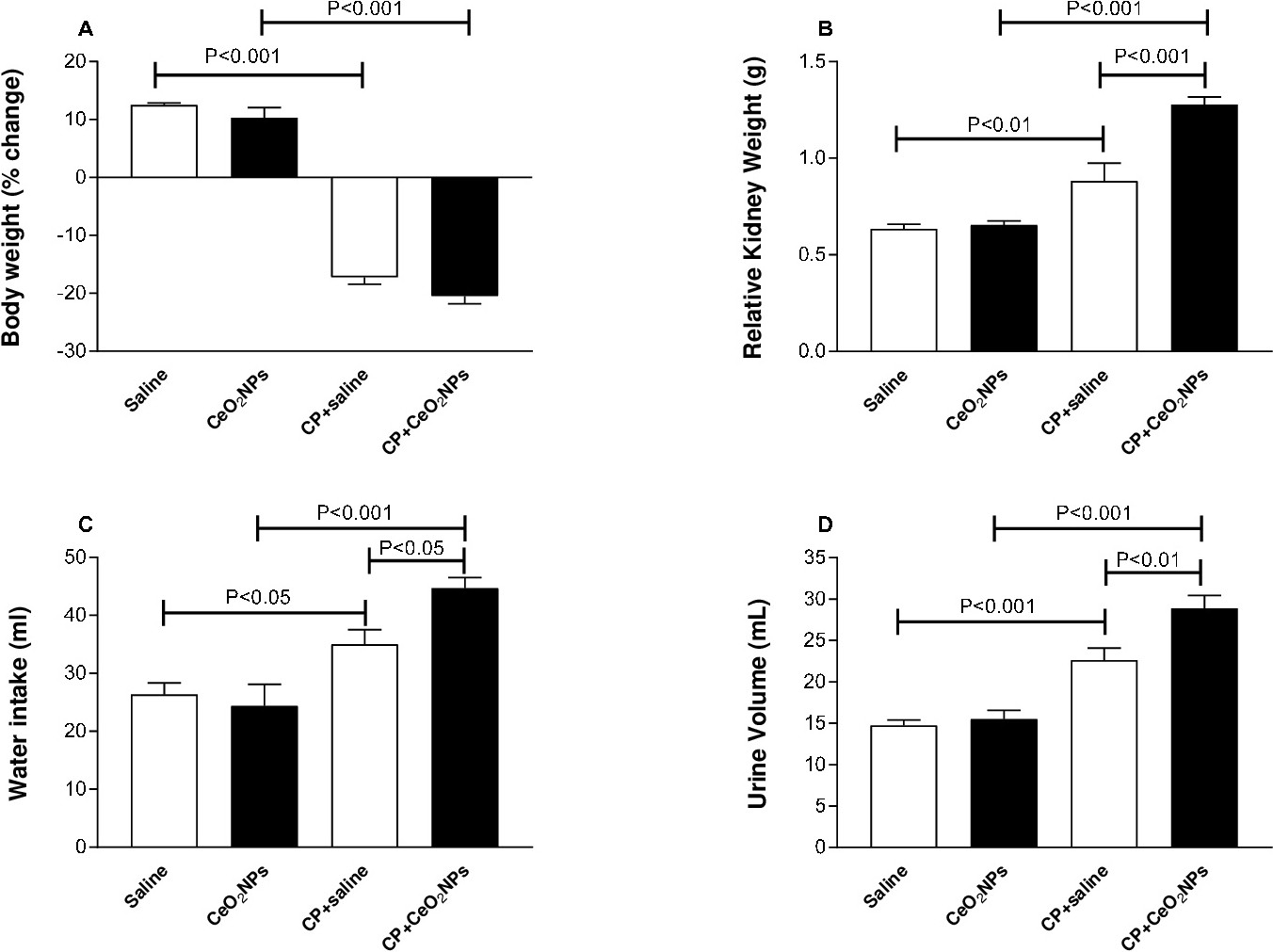Fig. 1. Body weight (A), relative kidney weight (B), water intake (C) and urine volume (D) in Wistar rats intratracheally instilled with either saline (control) or cerium oxide nanoparticles (CeO2 NPs) with or without cisplatin (CP) administration. Data are mean ± SEM. Body and kidney weights: saline (n=8), CeO2 NPs (n=8), CP+saline (n=8) and CP+ CeO2 NPs (n=8). Water intake: saline (n=6), CeO2 NPs (n=8), CP+saline (n=8) and CP+ CeO2 NPs (n=6). Urine volume: saline (n=8), CeO2 NPs (n=8), CP+saline (n=8) and CP+ CeO2 NPs (n=7).